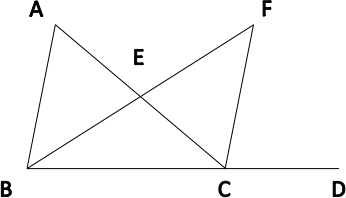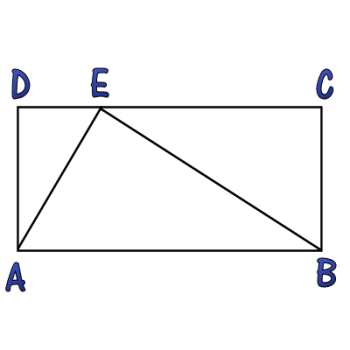Games
Problems
Go Pro!

# Angle Measure

Pro Problems > Math > Geometry > Triangles

## Angle Measure

Line segment AB is intersected by segment DC, with D on AB, between A and B.

In triangle ADC, m∠ADC = 30º + x and  m∠DAC = 50º

In triangle ABC, m∠ACB = 115º + x

In triangle CDB, m∠CDB = 140º + x

Find m∠CBD.

Presentation mode
Problem by Mr. Twitchell

## Solution

In order to make it feasible for teachers to use these problems in their classwork, no solutions are publicly visible, so students cannot simply look up the answers. If you would like to view the solutions to these problems, you must have a Virtual Classroom subscription.Assign this problem

## Similar Problems

### Triangle in a Square

A triangle is placed on the interior of a square so that two of the triangle's vertices lie on two adjacent vertices of the square, and the third vertex lies on the opposite side,
1
3
of the way between the two endpoints.

If the perimeter of the triangle (in linear units) is equal to the area of the triangle (in square units), what is the length of the side of the square?

### Three Two-Digit Angle Measures

The measures of the angles in a triangle are all two digit numbers, and two of them end in 3. Exactly two of them contain the digit 4. None of the angle measures are multiples of three. What are the measures of the angles?

### Two Triangles with Angle Measures

In a triangle, the angles measures are: 3x + 44, x, and 2x - 20. In a second triangle, two of the angle measures are x + 20 and 2x - 5. What is the measure of the third angle?

The angles in a triangle have measures x2 - 5, 2x + 18, and x + 37. What is the measure of the largest angle in the triangle?

### Angle Bisector

In the diagram shown,m ∠DCF = 80
m ∠ACF = 30
m ∠FBD = 50.

If segment BF bisects  ∠ABD, find m ∠BAC.

### Arithmetic Angles

The angles of a triangle are in arithmetic progression, and the difference between the largest and smallest angle is 42 degrees. What are the three angles?

### Forty-five and Thirty

Two of the angles of a triangle are 45 degrees and 30 degrees.  The altitude from the third angle has length 15 units. What is the triangle's area?

### Triangle and RectangleIn the diagram displayed, the triangle splits the rectangle into three similar triangles. Find the value of AD2 divided by the product of DE and EC.

### Three Triangles

The angles in a triangle have measures x, x + 10, and y.

The angles in a second triangle have measures x + y, 40, and 50.

The angles in a third triangle have measures x – y, 40, and z.

What is the value of z?

### Triangle Variables

An isosceles triangle is a triangle in which two of the angles have the same measure. In an isosceles triangle, the angle measures are 30 + x, 62 - x, and 10 + 2x + y. What are the possible numeric values for the measures of the angles?

Angles in a Triangle, Split Triangle, Angle Riddle, Triangle in a SquareLike us on Facebook to get updates about new resources